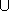#include <CGAL/QP_models.h>

### Definition

An object of class Quadratic_program_from_mps<NT> describes a convex quadratic program of the general form



 (QP) minimize xTDx+cTx+c0 subject to Ax ~ b, lxu

in n real variables x=(x0,...,xn-1). Here,

• A is an m × n matrix (the constraint matrix),
• b is an m-dimensional vector (the right-hand side),
•  ~  is an m-dimensional vector of relations from {, =,},
• l is an n-dimensional vector of lower bounds for x, where lj{-} for all j
• u is an n-dimensional vector of upper bounds for x, where uj{} for all j
• D is a symmetric positive-semidefinite n × n matrix (the quadratic objective function),
• c is an n-dimensional vector (the linear objective function), and
• c0 is a constant.

If D=0, the program is a linear program; if the variable bounds are x0, we have a nonnegative program.

The program data are read from an input stream in MPSFormat. This is a commonly used format for encoding linear and quadratic programs that is understood by many solvers. All values are expected to be readable into type NT. The constructed program can be further manipulated by using the set-methods below.

### Is Model for the Concepts

LinearProgram
NonnegativeLinearProgram

### Types

 Quadratic_program_from_mps::NT The number type of the program entries.

### Creation

 Quadratic_program_from_mps qp ( std::istream& in); reads qp from the input stream in.

### Operations

bool qp.is_valid () returns true if and only if an MPS-encoded quadratic program could be extracted from the input stream.

std::string qp.get_error () if !qp.is_valid(), this method returns an error message explaining why the input does not conform to the MPSFormat.

std::string qp.variable_name_by_index ( int j)
returns the name of the j-th variable.
 Precondition: j must not refer to a variable that has been added later, using one of the set methods below.

int qp.variable_index_by_name ( std::string name)
returns the index of the variable with name name. If there is no variable with this name, the result is -1.

std::string qp.constraint_name_by_index ( int i)
returns the name of the i-th constraint.
 Precondition: i must not refer to a constraint that has been added later, using one of the set methods below.

int qp.constraint_index_by_name ( std::string name)
returns the index of the constraint with name name. If there is no constraint with this name, the result is -1.

bool qp.is_linear () returns true if and only if qp is a linear program.

bool qp.is_nonnegative () returns true if and only if qp is a nonnegative program.

void qp.set_a ( int j, int i, NT val) sets the entry Aij in column j and row i of the constraint matrix A of qp to val. An existing entry is overwritten. qp is enlarged if necessary to accomodate this entry.

void qp.set_b ( int i, NT val) sets the entry bi of qp to val. An existing entry is overwritten. qp is enlarged if necessary to accomodate this entry.

void qp.set_r ( int i, CGAL::Comparison_result rel)
sets the entry  ~ i of qp to rel. CGAL::SMALLER means that the i-th constraint is of type ``'', CGAL::EQUAL means ``='', and CGAL::LARGER encodes ``''. An existing entry is overwritten. qp is enlarged if necessary to accomodate this entry.

void qp.set_l ( int j, bool is_finite, NT val = NT(0))
if is_finite, this sets the entry lj of qp to val, otherwise it sets lj to -. An existing entry is overwritten. qp is enlarged if necessary to accomodate this entry.

void qp.set_u ( int j, bool is_finite, NT val = NT(0))
if is_finite, this sets the entry uj of qp to val, otherwise it sets uj to. An existing entry is overwritten. qp is enlarged if necessary to accomodate this entry.

void qp.set_c ( int j, NT val) sets the entry cj of qp to val. An existing entry is overwritten. qp is enlarged if necessary to accomodate this entry.

void qp.set_c0 ( NT val) sets the entry c0 of qp to val. An existing entry is overwritten.

void qp.set_d ( int i, int j, NT val) sets the entries 2Dij and 2Dji of qp to val. Existing entries are overwritten. qp is enlarged if necessary to accomodate these entries.
 Precondition: j <= i